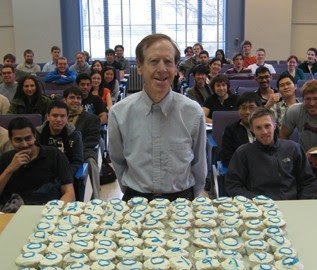# The Fundamental Theorem of Linear AlgebraThis is a series of articles devoted to Gilbert Strang’s Paper “The fundamental theorem of linear algebra” published by American Mathematical Monthly in 1993.

The paper describes the “Strang’s diagram”, a diagram that shows actions of $A$, an $m \times n$ matrix, as linear transformations from the space $\mathbb R^m$ to $\mathbb R^n$. The diagram helps to understand the fundamental concepts of Linear Algebra in terms of the four subspaces by visually illustrating the actions of $A$ on all these subspaces.In this series of blog posts we will work through the paper and will try to understand it.

There are 4 parts of the Fundamental theorem of Linear Algebra:

1. The dimensions of the subspaces;
2. The orthogonality of the subspaces;
3. The basis vectors are orthogonal;
4. The matrix with respect to these bases is orthogonal.

So there will be 4 blog posts based based on the paper:

1. The Four Fundamental Subspaces (theorem part 1 and part 2)
2. The Least Squares: Projection onto Subspaces (theorem part 2)
3. Singular Value Decomposition (theorem parts 3 and 4)
4. Pseudoinverse (theorem parts 3 and 4)

This series of blog posts is based on the report I wrote for the “Hot Topics in Information Management” course taught by Sebastian Schelter and Asterios Katsifodimos at TU Berlin in the winter semester of 2014-2015. During the course I was mentored by Moritz Schubotz.

You can access the pdf of my report at http://alexeygrigorev.com/projects/imsem-ws14-lina/imsem.pdf.

The course is a part of the IT4BI program I participate in, and if you would like to learn more about this program, you can check posts tagged with “IT4BI” on this website. For the detailed description of classes we took at TU Berlin, see the post “IT4BI: Distributed and Large-Scale Business Intelligence”.

Sources:

• Strang, Gilbert. “The fundamental theorem of linear algebra.” American Mathematical Monthly (1993): 848-855. (pdf)
• Strang, Gilbert. “The Four Fundamental Subspaces: 4 Lines.” (pdf)
• Strang, Gilbert. Linear Algebra and Its Applications. Brooks Cole, 1988.
• Strang, Gilbert. Linear Algebra course at MIT OpenCourseWare

Also you can see our post “Best Time to Learn Linear Algebra is Now!” for other good Linear Algebra resources.

#### 1 comment:

1.eagerly awaiting the remaining posts!